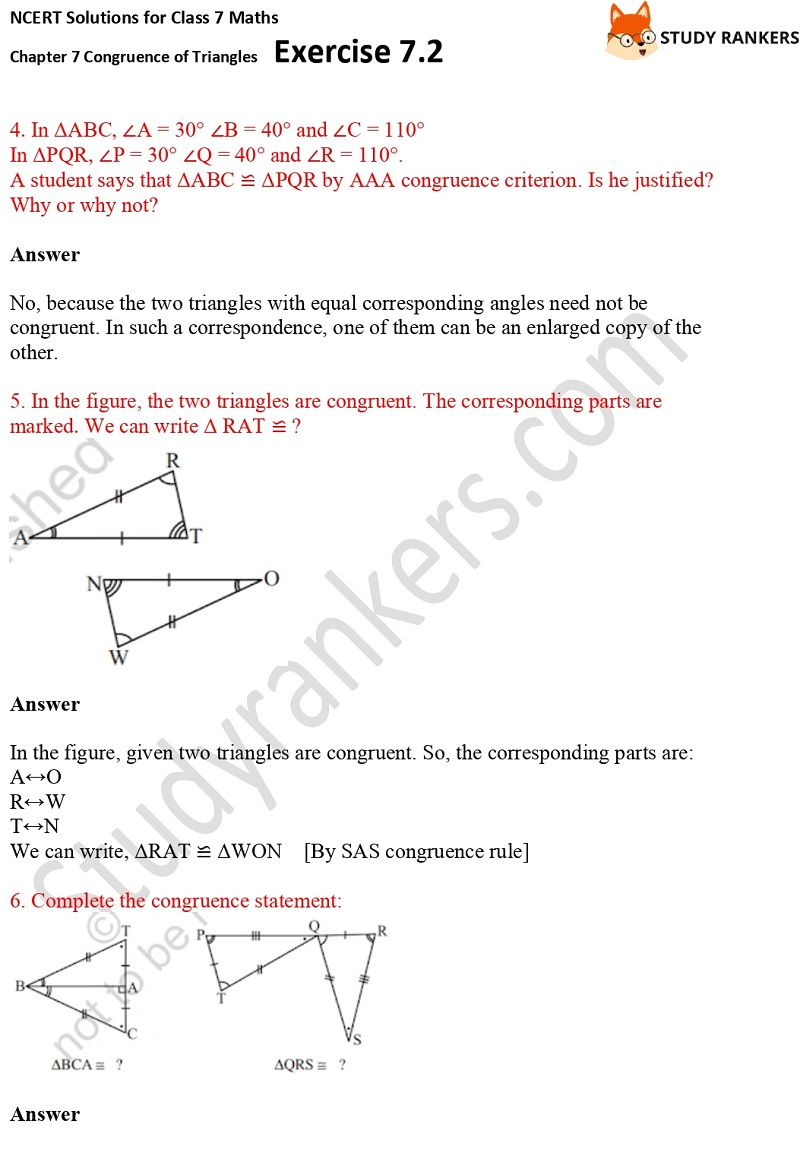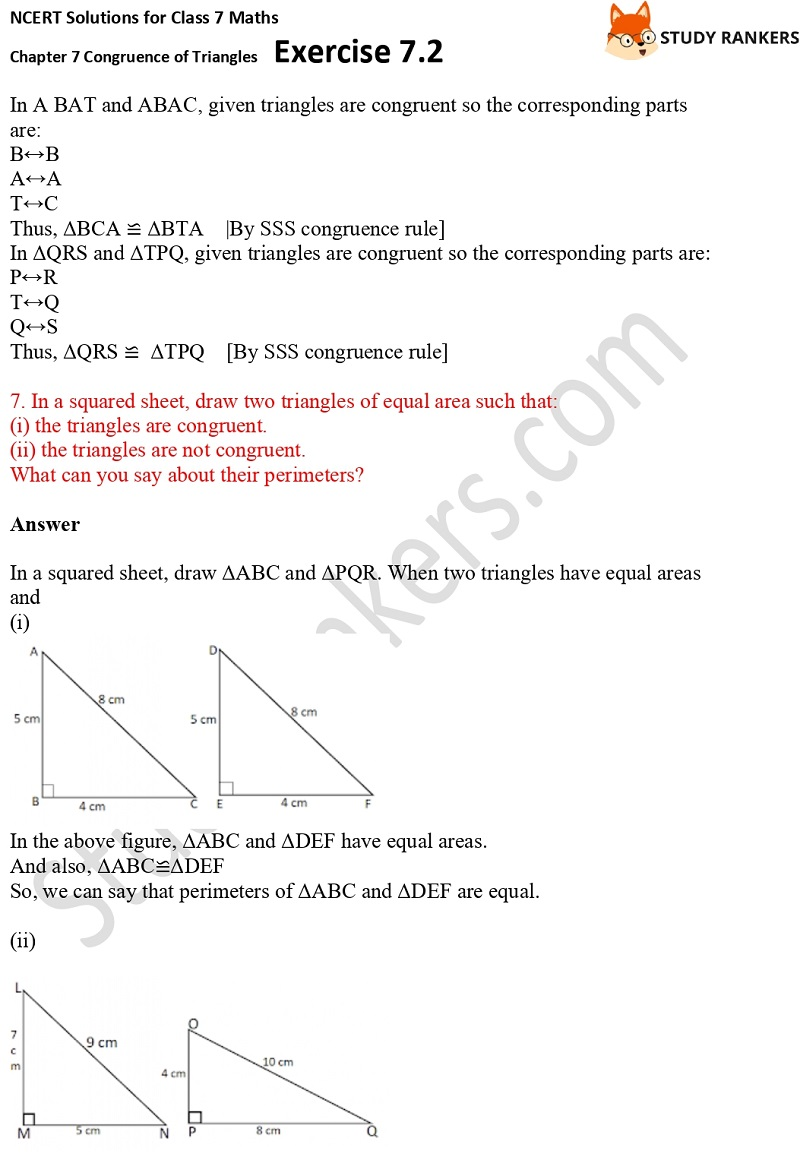>

# NCERT Solutions for Class 7 Maths Ch 7 Congruence of Triangles Exercise 7.2

Chapter 7 Congruence of Triangles Exercise 7.2 Class 7 Maths NCERT Solutions is helpful in completing homework in not time and the preparation of examinations. These NCERT Solutions are useful in clearing doubts and completing your homework on time and clear your doubts. NCERT Solutions for Class 7 Maths can help a students in knowing application of formulas to solve problems.

In Exercise 7.2, show that given two triangles are congruent.X